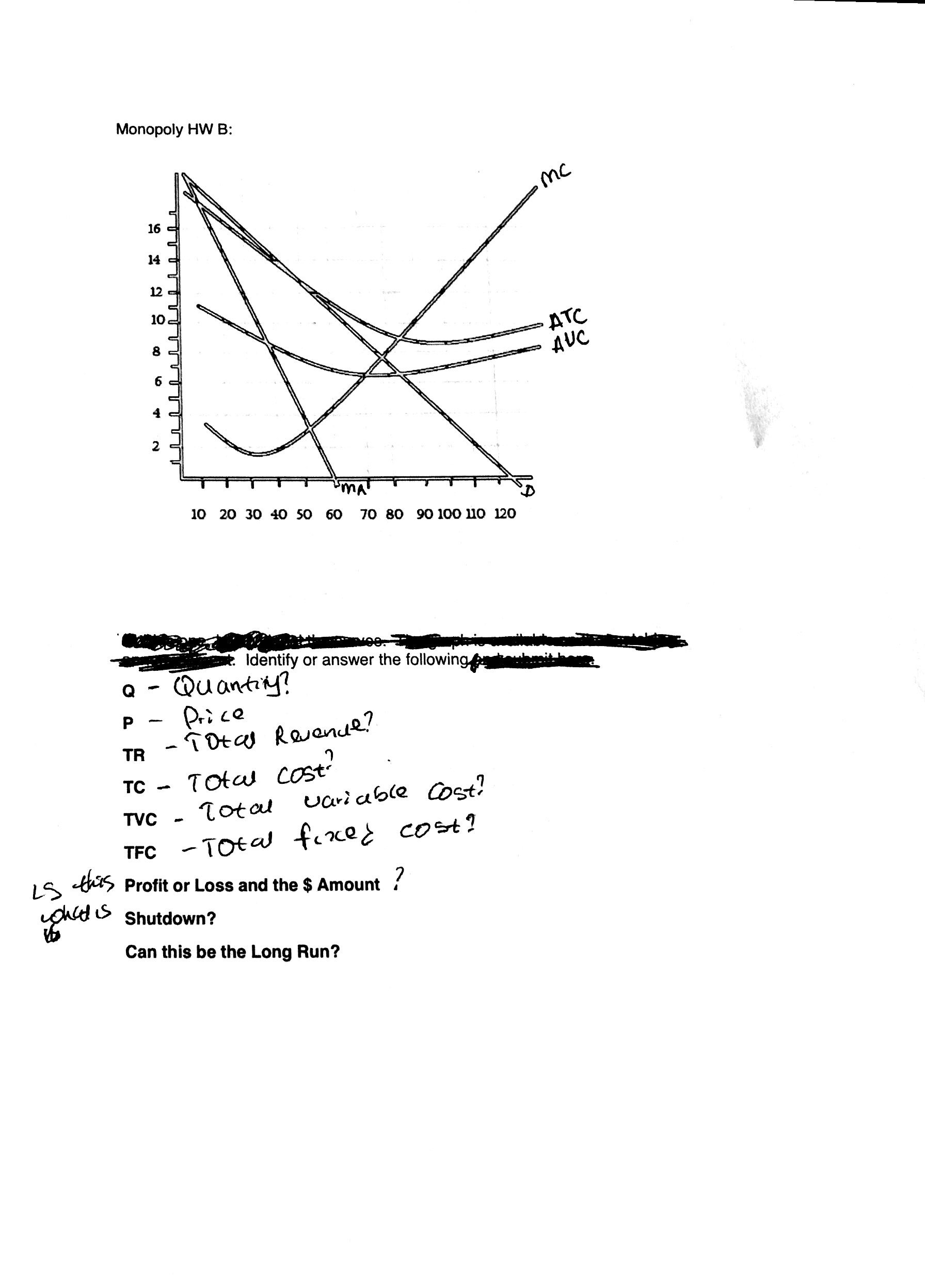Monopoly HW BMC1614 -12 -10ATCAUC8 =6 c4210 20 30 40 S0 6070 80 90 100 1о 120ldentifyCDuantor answer the followingP.TDtas Reanue?TRTota costTotalтCariable ostTVC- TOta fixe cost?TFCtiis Profit or Loss and the \$ Amount?LSShutdown?Can this be the Long Run?

Questionhelp_outlineImage TranscriptioncloseMonopoly HW B MC 16 14 - 12 - 10 ATC AUC 8 = 6 c 4 2 10 20 30 40 S0 60 70 80 90 100 1о 120 ldentify CDuant or answer the following P .TDtas Reanue? TR Tota cost Total тC ariable ost TVC - TOta fixe cost? TFC tiis Profit or Loss and the \$ Amount ? LS Shutdown? Can this be the Long Run? fullscreen
Step 1

In the aforementioned diagram, the downward sloping demand curve (D) and the marginal revenue (MR) curve are most important tools to determine the quantity of units produced and price charged by equating the marginal revenue curve with the marginal cost curve where the consumers’ willingness to pay (Price) will be determined by MR = MC and the ‘D’ curve. Thus, here, to maximize the profit 50 units will be produced at price \$13.

Now, determine total revenue (TR) by multiplying price and quantity, i.e.,

TR = Price * Quantity

= \$13 * 50

= \$650

Step 2

The total cost can be determined by multiplying minimum of ATC (Average Total Cost) with its respective quantity, i.e.,

TC = ATC * Q

= \$9 * 85

= \$765

Similarly, TVC (Total Variable Cost) is the product of AVC (Average Variable Cost) and Q (Quantity), i.e.,

TVC = AVC * Q

= \$6 * 70

= \$420

Further, TC = TFC + TVC, i.e.,

TFC = TC - TVC

= \$765 - \$420

= \$345

Step 3

In the short-run, there would be a loss in this market as TR < TC, i.e., \$650 < \$765. Hence, there is a loss of around \$115. However, the firm must not shutdown during this time period as in the long ...

Want to see the full answer?

See Solution

Want to see this answer and more?

Our solutions are written by experts, many with advanced degrees, and available 24/7

See Solution
Tagged in